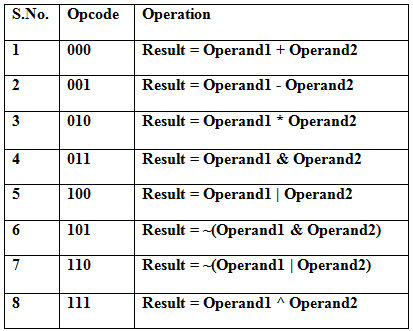1. ## Introduction

ALU is the fundamental building block of the processor, which is responsible for carrying out the arithmetic and logic functions. ALU comprises of combinatorial logic that implements arithmetic operations such as Addition, Subtraction and Multiplication,and logic operations such as AND, OR, NOT. The ALU gets operands from the register file or memory. The block diagram of a typical ALU is shown in Figure 1. The ALU reads two input operands In A and In B. The operation to perform on these input operands is selected using the control input Opcode. The ALU performs the selected operation on the input operands In A and In B and produces the output, Out. The ALU also updates different flag signals after performing the selected function. Note that the ALU is purely combinatorial logic and contains no registers or latches.Figure 1. Block diagram of 8-bit ALU

The arithmetic functions are much more complex to implement than the logic functions. The performance of the ALU depends upon the architecture of each structural components of the ALU. In this example only some basic ALU functions are implemented. The ALU is divided into an arithmetic section and a logical section.

The Arithmetic Unit compromises of three functions. They are:
• Subtraction
• Multiplication
The Logical Unit compromises of five functions. They are:
• Bitwise AND
• Bitwise OR
• Bitwise NAND
• Bitwise NOR
• Bitwise XOR
Flags: ALU updates the conditional flags, which are used by the processor to perform other operations like condition checking and branching. In this example two flags are implemented. They are:
• Zero - If all the bits of the result data are zero then the zero flag is set
• Carry out - If the addition of the two operands gives the carry out this flag is set

2. ## Truth Table

Figure 2 shows the truth table of the 8-bit ALU. The ALU works on 8-bit operands. It supports 8 instructions which are selected by the 3-bit Opcode.Figure 2. Truth table for 8-bit ALU.

3. ## Verilog Module

Figure 3 shows the Verilog module of the 8-bit ALU. The input to the ALU are 3-bit Opcode, and two 8-bit operands Operand1 and Operand2. The result of the operation is presented through the 16-bit Result port. In addition, there are two flags for carry (flagC) and zero (flagZ).Figure 3. Verilog module for 8-bit ALU

4. ## Verilog Code for the 8-bit ALU (ALU8bit.v)

 ``` `timescale 1ns / 1ps module ALU8bit( Opcode, Operand1, Operand2, Result, flagC, flagZ ); input [2:0] Opcode; input [7:0] Operand1, Operand2; output reg [15:0] Result = 16'b0; output reg flagC = 1'b0, flagZ = 1'b0; parameter [2:0] ADD = 3'b000, SUB = 3'b001, MUL = 3'b010, AND = 3'b011, OR = 3'b100, NAND = 3'b101, NOR = 3'b110, XOR = 3'b111; always @ (Opcode or Operand1 or Operand2) begin case (Opcode) ADD: begin Result = Operand1 + Operand2; flagC = Result; flagZ = (Result == 16'b0); end SUB: begin Result = Operand1 - Operand2; flagC = Result; flagZ = (Result == 16'b0); end MUL: begin Result = Operand1 * Operand2; flagZ = (Result == 16'b0); end AND: begin Result = Operand1 & Operand2; flagZ = (Result == 16'b0); end OR: begin Result = Operand1 | Operand2; flagZ = (Result == 16'b0); end NAND: begin Result = ~(Operand1 & Operand2); flagZ = (Result == 16'b0); end NOR: begin Result = ~(Operand1 | Operand2); flagZ = (Result == 16'b0); end XOR: begin Result = Operand1 ^ Operand2; flagZ = (Result == 16'b0); end default: begin Result = 16'b0; flagC = 1'b0; flagZ = 1'b0; end endcase end endmodule ``` Figure 4. Verilog code for 8-bit ALU

5. ## Verilog Test Bench for 8-bit ALU (ALU8bit_tb.v)

 ``` `timescale 1ns / 1ps module ALU8bit_tb; // Inputs reg [2:0] Opcode; reg [7:0] Operand1; reg [7:0] Operand2; // Outputs wire [15:0] Result; wire flagC; wire flagZ; //Temporary variable reg [2:0] count = 3'd0; // Instantiate the Unit Under Test (UUT) ALU8bit uut ( .Opcode(Opcode), .Operand1(Operand1), .Operand2(Operand2), .Result(Result), .flagC(flagC), .flagZ(flagZ) ); initial begin // Initialize Inputs Opcode = 3'b0; Operand1 = 8'd0; Operand2 = 8'd0; // Wait 100 ns for global reset to finish #100; // Add stimulus here Operand1 = 8'hAA; Operand2 = 8'h55; for (count = 0; count < 8; count = count + 1'b1) begin Opcode = count; #20; end end endmodule ``` Figure 5. Verilog Test-bench for 8-bit ALU

6. ## Timing DiagramFigure 6. Timing diagram of 8-bit ALU